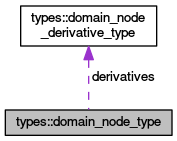OpenCMISS-Iron Internal API Documentation
types::domain_node_type Type Reference

Contains the topology information for a local node of a domain. More...

Collaboration diagram for types::domain_node_type:[legend]

## Public Attributes

integer(intg) local_number
The local node number in the domain. More...

integer(intg) mesh_number
The corresponding global node number in the mesh of the local node number in the domain i.e., the mesh node number. More...

integer(intg) global_number
The corresponding global number for the node i.e., the node number in the list of nodes for the region. More...

integer(intg) user_number
The corresponding user number for the node. More...

integer(intg) number_of_derivatives
The number of global derivatives at the node for the domain. Old CMISS name NKT(nj,np) More...

type(domain_node_derivative_type), dimension(:), allocatable derivatives
DERIVATIVES(derivative_idx) More...

integer(intg) number_of_surrounding_elements
The number of elements surrounding the node in the domain. Old CMISS name NENP(np,0,0:nr). More...

integer(intg), dimension(:), pointer surrounding_elements
SURROUNDING_ELEMENTS(nep). The local element number of the nep'th element that is surrounding the node. Old CMISS name NENP(np,nep,0:nr). More...

integer(intg) number_of_node_lines
The number of lines surrounding the node in the domain. More...

integer(intg), dimension(:), allocatable node_lines
NODE_LINES(nlp). The local line number of the nlp'th line that is surrounding the node. More...

integer(intg) number_of_node_faces
The number of faces surrounding the node in the domain. More...

integer(intg), dimension(:), allocatable node_faces
NODE_FACES(nlp). The local face number of the nlp'th face that is surrounding the node. More...

logical boundary_node
Is .TRUE. if the node is on the boundary of the mesh for the domain, .FALSE. if not. More...

## Detailed Description

Contains the topology information for a local node of a domain.

Definition at line 696 of file types.f90.

## Member Data Documentation

 logical types::domain_node_type::boundary_node

Is .TRUE. if the node is on the boundary of the mesh for the domain, .FALSE. if not.

Definition at line 709 of file types.f90.

 type(domain_node_derivative_type), dimension(:), allocatable types::domain_node_type::derivatives

DERIVATIVES(derivative_idx)

Definition at line 702 of file types.f90.

 integer(intg) types::domain_node_type::global_number

The corresponding global number for the node i.e., the node number in the list of nodes for the region.

Definition at line 699 of file types.f90.

 integer(intg) types::domain_node_type::local_number

The local node number in the domain.

Definition at line 697 of file types.f90.

 integer(intg) types::domain_node_type::mesh_number

The corresponding global node number in the mesh of the local node number in the domain i.e., the mesh node number.

Definition at line 698 of file types.f90.

 integer(intg), dimension(:), allocatable types::domain_node_type::node_faces

NODE_FACES(nlp). The local face number of the nlp'th face that is surrounding the node.

Definition at line 708 of file types.f90.

 integer(intg), dimension(:), allocatable types::domain_node_type::node_lines

NODE_LINES(nlp). The local line number of the nlp'th line that is surrounding the node.

Definition at line 706 of file types.f90.

 integer(intg) types::domain_node_type::number_of_derivatives

The number of global derivatives at the node for the domain. Old CMISS name NKT(nj,np)

Definition at line 701 of file types.f90.

 integer(intg) types::domain_node_type::number_of_node_faces

The number of faces surrounding the node in the domain.

Definition at line 707 of file types.f90.

 integer(intg) types::domain_node_type::number_of_node_lines

The number of lines surrounding the node in the domain.

Definition at line 705 of file types.f90.

 integer(intg) types::domain_node_type::number_of_surrounding_elements

The number of elements surrounding the node in the domain. Old CMISS name NENP(np,0,0:nr).

Definition at line 703 of file types.f90.

 integer(intg), dimension(:), pointer types::domain_node_type::surrounding_elements

SURROUNDING_ELEMENTS(nep). The local element number of the nep'th element that is surrounding the node. Old CMISS name NENP(np,nep,0:nr).

Todo:
Change this to allocatable.

Definition at line 704 of file types.f90.

 integer(intg) types::domain_node_type::user_number

The corresponding user number for the node.

Definition at line 700 of file types.f90.